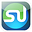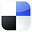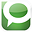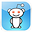# ANOVA TestDone in ExcelTo Improve Marketing

The 3 ANOVA functions in Excel can be real power tools for a marketer, if you know how to use them. Let’s take a look at what they can do for you. Below is a video that will show in detail how to use all 3 types of ANOVA that are built into Excel in order to improve your pay-per-click marketing campaigns. In the video, each of the 3 ANOVA types will be applied to the same data set. The video will demonstrate how the data is input, how the ANOVA is run, and how the output is interpretted for each ANOVA type. The article below also discusses the same information. Here is the video:

Step-By-Step Video Showing How To Improve Your Pay-Per-Click Marketing Using All 3 Types of ANOVA Built Into Excel

What Is ANOVA?

Marketers generally use ANOVA to tell them whether changing one element of a marketing campaign actually had an effect on the outcome. ANOVA testing should be applied only when that element being tested has at least three variations. For example, suppose you wanted to test whether a product’s color makes a difference in sales. To answer that question using ANOVA, your product would have to come in at least three colors to run an ANOVA test.

ANOVA, Analysis of Variance, tells the marketer whether or not the test output had enough variance in it to support the claim that varying the tested element actually did make a difference in the output.

ANOVA Output

As is common in most statistical problems, ANOVA’s output does not provide a definitive Yes or No answer but gives the probability that varying an input had an effect on the output. In ANOVA, this probability is called the Degree of Certainty. ANOVA testing requires a Degree of Certainty to be specified up front.

The ANOVA test answers the question of whether varying an element affected the output within a required degree of certainty. The output of an ANOVA might be explained as follows, “Yes, the variance observed in the output was large enough to conclude within 95% certainty that varying the element being tested did affect the output. We can reject the Null Hypothesis which stated that varying the tested element did not have effect on the output.”

The 3 Types of ANOVA Built Into Excel

The first type is called Single-Factor ANOVA. This is used to test only one input element. This is how the data must be arranged on the Excel spread sheet for this type analysis. The yellow-highlighted cells below are those that are selected as the input for Single-Factor ANOVA in Excel.

Excel Data Ready For Input - Single-Factor ANOVA
(Yellow-Highlighted Cells Are the Input Range)
Click On Image To See Enlarged View

Excel Output of Single-Factor ANOVA
Click On Image To See Enlarged View

The second type is called Two-Factor ANOVA Without Replication and tests two elements simultaneously. This is how the data must be arranged on the Excel spread sheet for this type analysis. The yellow-highlighted cells below are those that are selected as the input for Single-Factor ANOVA in Excel.

Two-Factor ANOVA Without Replication
(Yellow-Highlighted Cells Are the Input Range)
Click On Image To See Enlarged View

Excel Output of
Two-Factor ANOVA Without Replication
Click On Image To See Enlarged View

The third and final type of built-in ANOVA is called Two-Factor ANOVA With Replication. This ANOVA test simply replicates a two-factor test in at least two places in order to test whether interaction between the two factors also has an affect on the output.

Two-Factor ANOVA With Replication
(Yellow-Highlighted Cells Are the Input Range)
Click On Image To See Enlarged View

Excel Output of
Two-Factor ANOVA With Replication
Click On Image To See Enlarged View

In Excel, we are going to conduct one experiment and run the output of that experiment through all three types of ANOVA so you can observe the differences in how the data is inserted and how the Excel output is interpreted in each case. The above video shows the output of this experiment being run through all 3 ANOVA tests in Excel and how the different ANOVA outputs are interpreted.

The ANOVA Test We Ran

In a nutshell, the test is conducted as follows: we are testing ads in a pay-per-click campaign to determine whether varying the headline, ad text, or interaction between headline and ad text affects the click-through rate. Click-Through Rate (CTR) of an ad equals the number of ad impressions divided by the number of clicks that the ad generated.

We will use Excel to apply all three types of ANOVA testing to the same set of output data so that we can observe the differences in how data needs to be input into Excel for each ANOVA type and how the Excel output is interpreted for each case.

Single-Factor ANOVA will be applied to the output to determine if the varying the headlines affected CTR. Two-Factor ANOVA Without Replication will applied to the output to determine if varying headlines OR varying the ad text affected CTR. Two-Factor ANOVA With Replication will be applied to the output to determine whether variation in headlines OR ad text OR interaction between headlines and ad text affected CTR.

Once again. viewing the embedded video is probably the easiest way to quickly understand how data is input into Excel and how the Excel output is interpreted for each test.

In the video, all three types of Excel ANOVA are applied to the same set of output data.

Here Is a Summary of the Test That Was Run:

Step 1) Create 5 Sets of Ad Text For 1 Ad Group

5 different sets of ad text were created to be used within a single ad group. Ads within a single ad group should be tightly focused on a single theme, product, and landing page. We tried as much as possible for that to be the case here. One key to successful ANOVA testing to remove all other variation from the test except the specific elements being tested.

3 sets of headlines were created to be used in this ad group and were then combined with each of the 5 sets of ad text. This created a total of 15 possible combinations of ad text / headline.

Each of these 15 combinations of ad text / headline were run on both the Google and Yahoo pay-per-click search networks under similar conditions until 1 million ad impressions on each network were reached for each of the 15 ad text / headline combinations.

Step 4) Prepare the Test Results For Excel ANOVA

The output of this test was recorded as shown in the video. The linked video shows how output data must be recorded for insertion into Excel ANOVA. Each type of ANOVA in Excel requires the input data to be formatted slightly differently.

Step 5) Run All 3 Types of ANOVA On Test Results

Single-Factor ANOVA was applied to the output from Google to determine within 95% certainty whether varying the headline affects the output. Two-Factor ANOVA Without Replication was applied to the Google data to determine whether varying headline or ad text affects the output. Finally, Two-Factor ANOVA With Replication was applied to the output from both Google and Yahoo to determine whether varying headlines, ad text, or the interaction between headlines and ad text affect the output.

Step 6) Evaluate the Excel Output For Each ANOVA

The Excel output from each ANOVA test run in Excel was then interpreted. Each element being tested (headlines, ad text, and interaction) will have a separate P Value that will appear in the Excel output. The P Value for each tested element can be interpreted as being the probability of the observed variance in the output was due only to chance and not the result of varying the tested element.

Here is the output for the Single-Factor ANOVA test we ran:
Click On Image To See Enlarged View

Note that the P Value associated with Headlines = 0.001307, less that the Alpha of 0.05. We can therefore reject the Headlines Null Hypothesis and state with 95% certainty that varying the Headlines affected Click-Through Rate.

Here is the output for the Two-Factor ANOVA Without Replication test we ran:
Click On Image To See Enlarged View

Note that the P Value associated with Headlines (in Blue) is 0.00528, which is less that the Alpha of 0.05. However, the P Value associated with Ad Text (in yellow) is 0.627, which is greater than the Alpha of 0.05.

We can therefore reject the Headlines Null Hypothesis and state with 95% certainty that varying the Headlines affected the CTR. We cannot, howver, reject the Ad Text Null Hypothesis and we cannot state with 95% certainty that varying the Ad Text affected CTR.

Here is the output for the Two-Factor ANOVA With Replication test we ran:
Click On Image To See Enlarged View

Note that the P Value associated with Headlines (in Blue) is 1.18 E-06 which is less that the Alpha of 0.05. The P Value associated with Interaction Between Headlines and Ad Text (in Green) is 0.0402 which is less that the Alpha of 0.05. However, the P Value associated with Ad Text (in yellow) is 0.156, which is greater than the Alpha of 0.05.

We can therefore reject the Headlines Null Hypothesis and state with 95% certainty that varying the Headlines affected the CTR. We can also reject the Interaction Null Hypothesis and state with 95% certainty that varying the Interaction Between Headlines and Ad Text affected the CTR.   We cannot, howver, reject the Ad Text Null Hypothesis and we cannot state with 95% certainty that varying the Ad Text affected CTR.

Example of How To Interpret the P Value

For example, if the P Value associated with varying the headline has a value of 0.010, it means that there is only a 1% chance that the variance in the output could have occurred entirely by random chance and not by varying the headline. In other words, there is a 99% chance the varying the headline affected the output. If the degree of certainty that you required for this ANOVA test was 95%, then alpha equals 0.05 (1 – 95% = 0.05).

In the case that the P Value associated with headline equals 0.01, which is smaller than the alpha of 0.05, you can state that you are at least 95% certain that you can reject the Null Hypothesis which states that varying the headline had no effect on the output.

The Correct Interpretation of the ANOVA Test

The correct interpretation of an ANOVA is to state whether or not you can reject the Null Hypothesis (varying the element did not affect the output), not its corollary that you can now accept the Alternate Hypothesis (varying the element did affect the output).

The Big "However"....

However, we’re in business and not in statistics class, so if the P Value associated with an element is less than alpha, go ahead and state that varying the tested element did affect output within your required degree of certainty.

Once again, the embedded video above which will show you exactly how to setup, run, and interpret all three Excel ANOVA tests on the same set of pay-per-click ad data.

In a Nutshell, Use ANOVA in Excel To Find Out If Changes You Made To Your Marketing Campaigns Really Made a Difference.

If You Like This, Then Share It...Excel Master Series Blog Directory

Statistical Topics and Articles In Each Topic

• Histograms in Excel
• Bar Chart in Excel
• Combinations & Permutations in Excel
• Normal Distribution in Excel
• t-Distribution in Excel
• Binomial Distribution in Excel
• z-Tests in Excel
• t-Tests in Excel
• Hypothesis Tests of Proportion in Excel
• Chi-Square Independence Tests in Excel
• Chi-Square Goodness-Of-Fit Tests in Excel
• F Tests in Excel
• Correlation in Excel
• Pearson Correlation in Excel
• Spearman Correlation in Excel
• Confidence Intervals in Excel
• Simple Linear Regression in Excel
• Multiple Linear Regression in Excel
• Logistic Regression in Excel
• Single-Factor ANOVA in Excel
• Two-Factor ANOVA With Replication in Excel
• Two-Factor ANOVA Without Replication in Excel
• Creating Interactive Graphs of Statistical Distributions in Excel
• Solving Problems With Other Distributions in Excel
• Optimization With Excel Solver
• Chi-Square Population Variance Test in Excel
• Analyzing Data With Pivot Tables
• SEO Functions in Excel
• Time Series Analysis in Excel